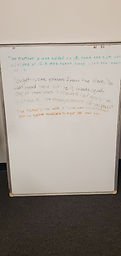Mr. Jake

Target 1​

Lesson Type:

New

Algebra

:

Variable

Solve mathematical problems by creating algebraic expressions.

1:

Create equations using a variable to explain real-world situations.

2:

Examine real-world problems and translate those patterns into algebraic expressions.

3:

Use variables to represent numbers and write expression when solving real-world mathematical problems.

4:

Use variable expressions to represent quantities that change in relationship to one another.

5:

Reason with quantities by constructing algebraic expressions.

7th

Vocabulary:

Variables, Equation, Order of Operations, Percent

Activities:

Solved problems involving variables

Created equations from word problems and solved for the unknown information.Home Exploration

Guiding Questions:Absent Students:

Target 2

:

1:

Construct a scatter plot from given data.

Vocabulary:

Activities:Home Exploration

Guiding Questions:Target 3

:

Vocabulary:

Activities:Home Exploration